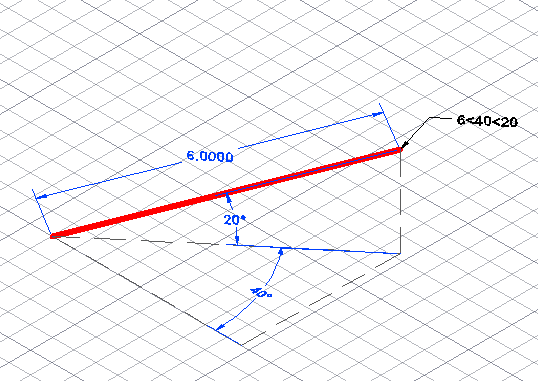The most comprehensive AutoCAD book around!# Use spherical coordinates in 3D AutoCAD drawings

Spherical coordinates are a way of specifying coordinates in 3D. Mostly, you use them with the LINE command. They’re good for finding a coordinate that you might use in a solid or surface. (I cover cylindrical coordinates, which are similar, here.)

Spherical coordinates have the format distance<angle<angle. An example would be 6<40<20. The 3 parts of the coordinate are as follows:

1. Distance: Units in the XY plane from the last point
2. Angle: Degrees from the X axis in the XY plane
3. Second angle: Degrees from the XY plane along the Z axis

Spherical coordinates can be absolute or relative. You don’t need the @ symbol to make relative coordinates if Dynamic Input is on and set to the default option of relative coordinates.

Here you see a red line drawn from 8,5 to 6<40<20. Note that the length of the line is the same as the distance in the coordinate (unlike cylindrical coordinates).Do you use spherical units? When do you find them useful?

•Rolland Grigsby

•Stijn Tas
•Luisa